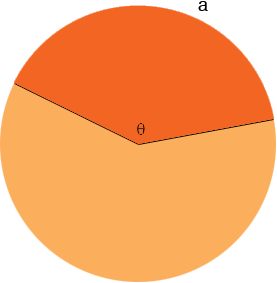SEARCH HOMEMath Central Quandaries & QueriesQuestion from sara, a student: Hi! The area of a sector of a circle is 25 sq cm. The radius of the sector is 6 cm. Calculate the perimeter of the sector. Many thanks!!!Sara,

The perimeter of a sector is composed of three pieces, an arc of the circle and two radii. You know the length of the radii so what remains is to find the length of the arc.Suppose the length of the arc is a cm and the angle at the centre of the circle subtended by the arc is θ radians. The area of the sector is then

A = 1/2 r2 θ

and the length of the arc is

a = r θ

This is enough information to find a.

PennyMath Central is supported by the University of Regina and The Pacific Institute for the Mathematical Sciences.